# Triangle + rectangle - math problems

#### Number of problems found: 79

• Diagonal - simple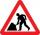Calculate the length of the diagonal of a rectangle with dimensions 5 cm and 12 cm.
• Acreage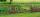What acreage has a rectangular plot whose diagonal is 34 meters long and one side has a length of 16 meters. ...
• CarpetThe room is 10 x 5 meters. You have the role of carpet width of 1 meter. Make rectangular cut of roll that piece of carpet will be longest possible and it fit into the room. How long is a piece of carpet? Note .: carpet will not be parallel with the diago
• Octagon from rectangleFrom tablecloth rectangular shape with dimensions of 4 dm and 8 dm we cuts down the corners in the shape of isosceles triangles. It thus formed an octagon with area 26 dm2. How many dm2 we cuts down?
• Circular poolThe base of the pool is a circle with a radius r = 10 m, excluding a circular segment that determines the chord length 10 meters. The pool depth is h = 2m. How many hectoliters of water can fit into the pool?
• Triangular prismCalculate the surface area and volume of a triangular prism, base right triangle if a = 3 cm, b = 4 cm, c = 5 cm, and height of prism h=12 cm.
• Again saw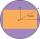From the trunk of the tree we have to a sculpture beam with rectangular cross-section with dimensions 146 mm and 128 mm. What is the trunk smallest diameter?
• Triangular prism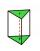The base perpendicular triangular prism is a right triangle whose hypotenuse measures 5 cm and one cathetus 2 cm. Height of the prism is equal to 7/9 of the perimeter of the base. Calculate the surface area of prism.
• BoxCardboard box-shaped quadrangular prism with a rhombic base. Rhombus has a side 5 cm, and one diagonal 8 cm long, and the box's height is 12 cm. The box will open at the top. How many cm2 of cardboard do we need to cover overlap and joints that are 5% of
• Inscribed rectangle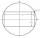The circle area is 216. Determine the area of inscribed rectangle with one side 5 long.
• RectangleCalculate the length of the side GN and diagonal QN of rectangle QGNH when given: |HN| = 25 cm and angle ∠ QGH = 28 degrees.
• Block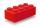Calculate the volume of a cuboid ABCDEFGH if |AB| = 16 cm, |BC| = 19 cm and the angle ∠CDG = 36.9°
• DiagonalCalculate the diagonal length of the rectangle ABCD with sides a = 8 cm, b = 7 cm.
• RectangleThe rectangle is 21 cm long and 38 cm wide. Determine the radius of the circle circumscribing rectangle.
• RectangleIn rectangle ABCD with sides, |AB|=19, |AD|=16 is from point A guided perpendicular to the diagonal BD, which intersects at point P. Determine the ratio ?.
• DiagonalDetermine the dimensions of the cuboid, if diagonal long 53 dm has an angle with one edge 42° and with another edge 64°.
• RectangleIn a rectangle with sides, 8 and 9 mark the diagonal. What is the probability that a randomly selected point within the rectangle is closer to the diagonal than to any side of the rectangle?
• Median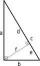In the right triangle are sides a=96 dm b=31 dm. Calculate the length of the medians tc to the hypotenuse.
• Widescreen monitorComputer businesses hit by a wave of widescreen monitors and televisions. Calculate the area of ​​the LCD monitor with a diagonal size 20 inches at ratio 4:3 and then 16:9 aspect ratio. Is buying widescreen monitors with the same diagonal more advantageou

Do you have an exciting math question or word problem that you can't solve? Ask a question or post a math problem, and we can try to solve it.

We will send a solution to your e-mail address. Solved examples are also published here. Please enter the e-mail correctly and check whether you don't have a full mailbox.